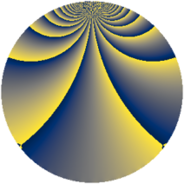# Properties

 Label 2850.2.bdLevel $2850$ Weight $2$ Character orbit 2850.bd Rep. character $\chi_{2850}(1493,\cdot)$ Character field $\Q(\zeta_{12})$ Dimension $480$ Sturm bound $1200$

# Learn more about

## Defining parameters

 Level: $$N$$ $$=$$ $$2850 = 2 \cdot 3 \cdot 5^{2} \cdot 19$$ Weight: $$k$$ $$=$$ $$2$$ Character orbit: $$[\chi]$$ $$=$$ 2850.bd (of order $$12$$ and degree $$4$$) Character conductor: $$\operatorname{cond}(\chi)$$ $$=$$ $$285$$ Character field: $$\Q(\zeta_{12})$$ Sturm bound: $$1200$$

## Dimensions

The following table gives the dimensions of various subspaces of $$M_{2}(2850, [\chi])$$.

Total New Old
Modular forms 2496 480 2016
Cusp forms 2304 480 1824
Eisenstein series 192 0 192

## Trace form

 $$480q + O(q^{10})$$ $$480q + 16q^{13} + 240q^{16} - 32q^{18} + 8q^{22} - 24q^{27} + 16q^{31} - 12q^{33} + 16q^{37} + 32q^{42} + 40q^{43} - 44q^{51} - 16q^{52} + 92q^{57} + 8q^{61} - 28q^{63} + 44q^{66} + 24q^{67} - 16q^{72} - 64q^{73} - 8q^{76} - 16q^{78} + 232q^{87} - 16q^{88} - 40q^{91} + 20q^{93} + 16q^{97} + O(q^{100})$$

## Decomposition of $$S_{2}^{\mathrm{new}}(2850, [\chi])$$ into newform subspaces

The newforms in this space have not yet been added to the LMFDB.

## Decomposition of $$S_{2}^{\mathrm{old}}(2850, [\chi])$$ into lower level spaces

$$S_{2}^{\mathrm{old}}(2850, [\chi]) \cong$$ $$S_{2}^{\mathrm{new}}(285, [\chi])$$$$^{\oplus 4}$$$$\oplus$$$$S_{2}^{\mathrm{new}}(570, [\chi])$$$$^{\oplus 2}$$$$\oplus$$$$S_{2}^{\mathrm{new}}(1425, [\chi])$$$$^{\oplus 2}$$